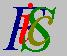Mauro Murzi's pages on Philosophy of Science - Quantum mechanics
Geometrical representation of complex numbers

# [2. Mathematical elements.]

## Geometrical representation of complex numbers.

Complex numbers can be geometrically represented on the two dimensional Cartesian coordinate system, assigning the real part to the x-axis and the imaginary part to the y-axis. The complex number z=a+ib is represented as the vector from the origin to the point (a,b). In this geometrical interpretation of complex numbers some operations have an intuitive meaning.

• The module of a complex number is the length of the vector representing the complex number.
• The addition of two complex numbers is the analogous of the sum of the corresponding vectors.
• The multiplication of a real number and a complex number is the analogous of multiplying the vector corresponding to the complex number by a scalar.

The following formula, known as Euler's formula, defines an interesting relation between complex numbers and trigonometric functions:  i x = cos + i sin x.